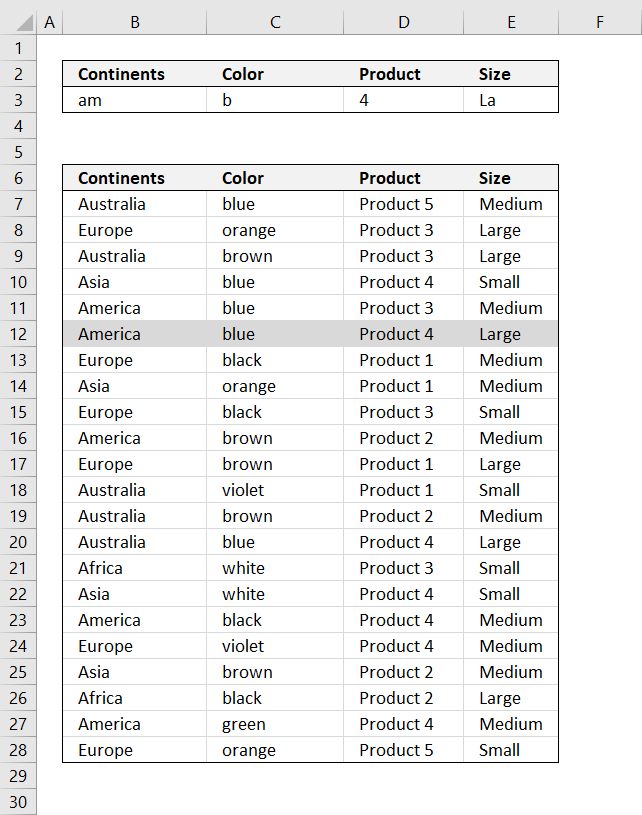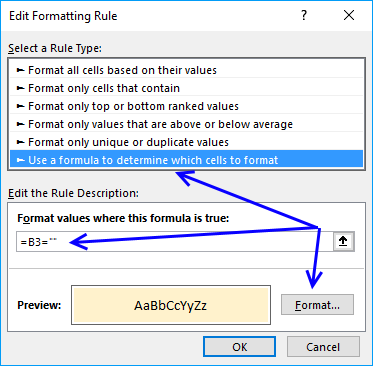Author: Oscar Cronquist Article last updated on January 15, 2019The picture above shows you how to highlight rows containing text strings using conditional formatting.

Example, continents criterion (cell B3) is only searched in column Continents (B7:B28). Color criterion is searched for in column Color, and so on.

Conditional formatting formula:

=COUNT(SEARCH(\$B\$3, \$B7)+1/(\$B7<>""))*COUNT(SEARCH(\$C\$3, \$C7)+1/(\$C7<>""))*COUNT(SEARCH(\$D\$3, \$D7)+1/(\$D7<>""))*COUNT(SEARCH(\$E\$3, \$E7)+1/(\$E7<>""))

The formula is not case sensitive. Replace SEARCH function with FIND to make it case sensitive.

Note: Conditional formatting is volatile meaning when the worksheet is recalculated all conditionally formatted cells are recalculated, this may slow down your worksheet considerably.

### How to apply conditional formatting formula

1. Select cells B6:E27
2. Press with left mouse button on "Home" tab
3. Press with left mouse button on "Conditional Formatting" button
4. Press with left mouse button on "New Rule.."
5. Press with left mouse button on "Use a formula to determine which cells to format"
6. Type above formula in "Format values where this formula is TRUE" window.7. Press with left mouse button on "Format.." button
8. Press with left mouse button on "Fill" tab
9. Select a color for highlighted cells.
10. Press with left mouse button on "Ok"
11. Press with left mouse button on "Ok"
12. Press with left mouse button on "Ok"

### How the conditional formatting formula works in cell B12

Note, the explanation below is for cell B12. Cell B12 is chosen because it is highlighted by the conditional formatting formula.

Step 1 - Understand relative and absolute cell references

A cell reference may or may not have dollar signs in front of the reference, it indicates the cell reference is fixed meaning it won't change when the cell is copied to other cells.

Example, cell reference \$D3 is fixed to column D (absolute cell reference), however, row number 3 changes (relative cell reference) when the cell is copied to another cell.

Note, an absolute cell reference (fixed) changes if you insert/delete a row or column.

Step 2 - Search cells to see if criteria are found

The SEARCH function returns the number of the character at which a specific character or text string is found reading left to right (not case-sensitive).

=COUNT(SEARCH(\$B\$3, \$B7)+1/(\$B7<>""))*COUNT(SEARCH(\$C\$3, \$C7)+1/(\$C7<>""))*COUNT(SEARCH(\$D\$3, \$D7)+1/(\$D7<>""))*COUNT(SEARCH(\$E\$3, \$E7)+1/(\$E7<>""))

becomes

=COUNT(SEARCH("am", \$B12)+1/(\$B12<>""))*COUNT(SEARCH("b", \$C12)+1/(\$C12<>""))*COUNT(SEARCH("4", \$D12)+1/(\$D12<>""))*COUNT(SEARCH("LA", \$E12)+1/(\$E12<>""))

becomes

=COUNT(SEARCH("am", "America")+1/("America"<>""))*COUNT(SEARCH("b", "blue")+1/("blue"<>""))*COUNT(SEARCH("4", "Product 4")+1/("Product 4"<>""))*COUNT(SEARCH("LA", "Large")+1/("Large"<>""))

becomes

=COUNT(1+1/("America"<>""))*COUNT(1+1/("blue"<>""))*COUNT(9+1/("Product 4"<>""))*COUNT(1+1/("Large"<>""))

Step 3 - Identify empty criteria and return TRUE or FALSE

The COUNT function counts all numerical values in an argument.

=COUNT(1+1/("America"<>""))*COUNT(1+1/("blue"<>""))*COUNT(9+1/("Product 4"<>""))*COUNT(1+1/("Large"<>""))

becomes

=COUNT(1+1/(TRUE))*COUNT(1+1/(TRUE))*COUNT(9+1/(TRUE))*COUNT(1+1/(TRUE))

becomes

=COUNT(1+1)*COUNT(1+1)*COUNT(9+1)*COUNT(1+1)

becomes

=COUNT(2)*COUNT(2)*COUNT(10)*COUNT(2)

and returns 1 (TRUE). Row 12 is highlighted

### Get the Excel filequickly-highlight-records-in-a-list-search-function-AND-logic.xlsx